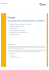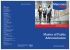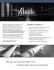# SE2 Structural Materials Final Exam For each problem, choose one

## Transcription

SE2 Structural Materials Final Exam For each problem, choose one
```SE2 Structural Materials
Final Exam
For each problem, choose one and only one most relevant answer on a Scantron sheet.
1. Which of the following statement is true:
(A) Ceramics are usually stiff and strong
(B) Polymers are usually stiff and strong
(C) Metals are usually stiff and brittle
(D) Composites are usually stiff and brittle
2. If a material is stiff and strong, it
(A) must also be tough
(B) must not be tough
(C) has a high yield strength
(D) has a low ultimate tensile strength (UTS)
3. To mass-produce long polymer pipes, a cost-efficient way is
(A) Extrusion
(B) Injection molding
(C) Friction welding
(D) Forging
4. Which of the following is NOT a surface treatment method:
(A) Polishing
(B) Coating
(C) Painting
(D) Quenching
5. The more fuel efficient, the better an aircraft would be. This statement specifies _______
for the design of aircraft.
(A) A constraint
(B) An objective
(C) a free variable
(D) None of the above
6. We are selecting the material for a new horizontal bar in a gym. The deflection of the
horizontal bar should not be too large or too small. The cross-sectional size should fit
with people’s hands. The cost should be minimized. Denote the cross-sectional area as A,
the cross-sectional radius as r, the Young’s modulus as E, the length as L, the density as
ρ, the geometrical factor as C1, and the material cost per mass as Cm. Which one of the
following gives the objective(s):
(A) C1EA2/4πL3
(B) ALρ Cm
(C) ALρ
(D) Both A and B
7. In the above problem, which one of the following gives a constraint:
(A) C1EA2/4πL3
(B) C1πEr4/4L3
(C) ALρ Cm
(D) Both A and B
8. In the above problem, to minimize the cost and to meet all the other requirements, we
need to maximize:
(A) EρCm
(B) E1/2ρCm
(C) E1/3ρCm
(D) 1/Cm
9. For the above problem, among the following materials, _________ is the best candidate:
(A) Wood (// grain)
(B) Stainless steel
(C) Glass fiber reinforced polymer (GFRP)
(D) Teflon (PTFE)
10. John and Johnna stand on the soil in the backyard of their house, both wearing hard, flatbottom shoes. The areas of their shoe bottoms are 100 cm2 and 80 cm2, respectively. John
weighs 140 lbs and Johnna weighs 90 lbs. They notice that John leaves deep footmarks in
the soil, while Johnna does not. Which one of the following is a possible value of the
yield strength of the soil:
(A) 80 kPa
(B) 60 kPa
(C) 30 kPa
(D) 20 kPa
11. Dislocations can move
(A) along any direction
(B) along any crystalline plane
(C) only along a certain directions in a certain crystalline planes
(D) only in a certain crystalline planes but can be along any direction in these planes
12. When a shear stress is applied, _________ can slide.
(A) only screw dislocations
(B) edge dislocations parallel to the stress
(C) edge dislocations normal to the stress
(D) only edge dislocations
13. Precipitation hardening may be more efficient than solution hardening, because
(A) the slip resistance of precipitation particles is higher than that of solute atoms
(B) additional resistance to dislocation sliding is offered by solute atoms
(C) precipitation phase distributes more uniformly
(D) the material has not been hardened
14. The more dislocations that a material has,
(A) the weaker the material would be
(B) the stronger the material would be
(C) the more grain boundary the material would have
(D) none of the above
15. Usually, the compression strength of a ceramic material is lower than its tensile strength.
This statement is
(A) True
(B) False
16. The initial length and diameter of a polymer bar are 10 cm and 10 mm, respectively. The
polymer bar is subjected to a tensile load of 1000 N, and the total elongation is 4 mm. Its
Young’s modulus is 10 GPa. The plastic strain is ___________
(A) 4%
(B) 0.13%
(C) 2.13%
(D) 3.87%
17. A beam has a circular cross section, and its two ends are simply supported. A
concentrated force is applied at the middle point. It is required that when the force is F0,
no plastic yielding should happen, and the deflection should be as small as possible. The
diameter of the beam must be equal to r0. Which of the following helps reach the design
objective?
(A) The cross-sectional radius, r, is reduced.
(B) The Young’s modulus, E, is increased.
(C) The yield strength of the material, σy, is reduced.
(D) All of the above.
18. In the above problem, for materials selection, the design index should be
(A) E/σy3
(B) E/σy4/3
(C) E/σy1/3
(D) E/σy
19. In the above problem, if the beam length must be equal to a certain value, L0, while the
beam diameter is flexible, for the materials selection, the design index should be
(A) E/σy3
(B) E/σy4/3
(C) E/σy1/3
(D) E/σy
20. In the above problem, when the beam length can be flexible while the beam diameter is
fixed, among the following materials ______ is the best candidate:
(A) Concrete
(B) Soda glass
(C) Wood
(D) Polyurethane (PU)
21. In the above problem, when the beam length is specified while the beam diameter is
flexible, among the following materials __________ is the best candidate:
(A) Brick
(B) Glass fiber reinforced polymer (GFRP)
(C) Teflon (PTFE)
(D) Wood
22. The fracture toughness of a material is 40 MPa⋅m1/2 and its stiffness is 100 GPa. In order
to break this material to form 1 m2 of new surface area, at least __________ of work must
be done.
(A) 16 J
(B) 40 J
(C) 16 kJ
(D) 40 kJ
23. A small piece of a precious alloy is harvested from a large structure, close to the tip of a
10 cm long surface crack. The structure worked under a tensile stress of 10 MPa. The
geometric constant (Y) can be taken as 1. The strength of the material is 70 MPa. What
should be the minimum distance between the small piece and the crack tip, so that the
harvested material does not have any permanent damage?
(A) 1 mm
(B) 2 mm
(C) 4 mm
(D) 8 mm
24. Interior wall insulation should insulate well, meaning low thermal conductivity, λ , but
require as little heat as possible to warm up when the central heating system is turned on
(if the wall absorbs heat the room stays cold). Which one of the following can be the best
candidate?
(A) Concrete
(B) Steel
(C) Cork
(D) Flexible polymer foam
25. There is a triple point at 0.01 C in the pressure-temperature equilibrium phase diagram
for pure water. What is the degree of freedom at the triple point at 0.01 oC?
(A) 0
(B) 1
(C) 2
(D) 3
26. According to the S-N curve on the right, the endurance limit of the steel is nearly
(A) 14 ksi
(B) 29 ksi
(C) 109 cycles
(D) 107 cycles
27. Refer to the S-N curves
on the right. If a structural
aluminum must work for
more than 200,000
known that the minimum
load (σmin) is 10 ksi, the
should not exceed
(A) 40 ksi
(B) 70 ksi
(C) 45 ksi
(D) 80 ksi
28. A flat metal plate is subjected to a fatigue loading. The maximum load is 100 MPa and
the R-ratio is 0. The critical stress intensity factor is 40 MPa⋅m1/2. What is the maximum
crack length that the plate can have without causing catastrophic failure? Assume the
geometric factor, Y, is 1.
(A) 51 mm
(B) 31 mm
(C) 21 mm
(D) 11 mm
29. In the above problem, a detailed study shows that the average grain size of the material is
10 microns, and the dislocation density is 106 per mm2. The width of the plate is 0.5 m
and the thickness is 0.1 m. The length of the plate is 0.8 m. Through visual inspection and
supersonic inspection, no defects are detected. We may assume that
(A) There are no cracks in the plate.
(B) There are microcracks in the plate, with the size around 0.1 m.
(C) There are microcracks in the plate, with the size around 10 microns.
(D) The dislocations are the precracks.
30. In the above problem, assume m = 1.7 and A = 6×10-12 in MPa and meter units. How
(A) 77.3 thousands
(B) 7.73 million
(C) 77.3 million
(D) 0.773 billion
31. The figure on the right shows the
creep behavior of a material.
Usually, the creep rate is measured
from Section
(A) OA
(B) AB
(C) BC
(D) CD
32. Refer to the L-M parameter curves
on the right. Equiaxed MAR-M 247
MFB alloy was employed to work at
700 oC under a constant load. It
worked for 1020 h before creep
failure happened. What is the creep
(A) 380 MPa
(B) 310 MPa
(C) 290 MPa
(D) 230 MPa
33. We need to choose the material for a
Trampoline. For given body weight
and jumping height, it must deform
as much as possible without fracture.
The most appropriate materials
performance index should be
(A) σy
(B) KIC
(C) KIC2/E
(D) KIC/E
34. In the above problem, among the following choices, _________ is LEAST attractive:
(A) steel
(B) leather
(C) carbon fiber reinforced polymers (CFRP)
(D) glass fiber reinforced polymers (GFRP)
35. We need to select the material for a safety pin, which can deform plastically when the
load exceeds 40 MPa to better seal the structure. The pin must support as much load as
possible without fracture. Among the following choices, _______ is most attractive:
(A) stainless steel
(B) some copper (Cu) alloys
(C) polypropylene (PP)
(D) some aluminum (Al) alloys
36. A copper block slides along a rough concrete surface. The block is cubic, with the size of
100 mm. After the copper block slides for 100 m, its weight is reduced by 5%. What is
the specific wear rate, Ω?
(A) 450
(B) 5×10-5
(C) 5×10-4
(D) 4.5×10-2
37. We need to assess the friction force between two materials, but do not have any data or
information of them. Among the following, what is the best we can do?
(A) Assume the friction coefficient is 0.5
(B) Assume there is no friction between them
(C) Assume the friction force equals to the pressure between the two materials
(D) Assume the friction force equals to 1/10 of the pressure between the two materials
38. Which of the following dose NOT involve direct contact of two solid materials;
(A) abrasive wear
(B) hydrodynamic lubrication
(D) boundary lubrication
39. A shaft rotates at the rate of 100 rpm. Its
diameter is 200 mm, and it is supported by
a bearing, via boundary lubrication. The
safe operation zone is shown in the figure
on the right (the same as the one given in
the lecture slides). What is the approximate
maximum bearing pressure for safe use?
(A) 1 MPa
(B) 3 MPa
(C) 30 MPa
(D) No enough information
40. The specific heat of a material is 0.4 J/gK. Which one of the following is the best
assessment of its density?
(A) 50 g/cc
(B) 25 g/cc
(C) 5 g/cc
(D) 0.5 g/cc
41. In a material, phonons travel at the speed of sound. Thus, the heat transfer also happens at
the speed of sound. This statement is
(A) True
(B) False
42. A 100 long rod is made of an unknown metal. It is known that if temperature reaches
800 oC it will melt. As temperature increases from room temperature (20 oC) to 220 oC,
what is the best assessment of the elongation of the rod?
(A) 0.50”
(B) 0.37”
(C) 0.24”
(D) 0.11”
43. A bimetallic temperature sensor consists of two layers of different metallic materials. As
temperature changes, it would deform. To maximize the temperature sensitivity, which
pair of the following choices are most attractive/
(A) Al alloy and Zn alloy
(B) Cu alloy and stainless steel
(C) Cu alloy and Ni alloy
(D) Mg alloy and W alloy
44. Which one of the following is
correct: in winter, copper feels
colder than wood, because
(A) copper has higher thermal
conductivity and thermal
diffusivity
(B) copper has a lower thermal
diffusivity but a higher thermal
diffusivity
(C) the temperature of copper is
indeed lower
(D) copper has a higher thermal
conductivity but a lower thermal
diffusivity
45. The phase diagram of pure iron is
shown above. What is the degree of freedom of the system when temperature is 2000 C
and pressure is 1 atm?
(A) 3
(B) 2
(C) 1
(D) 0
46. According to the phase diagram of iron, solid iron always melts at 1538 C, no matter
what the pressure is. This statement is
(A) True
(B) False
47. The phase
diagram of Cu-Ni
alloy is shown on
the right. For an
alloy contains 50
wt% of Ni, at
1300 C, what are
the phases in the
system?
(A) Liquid phase
(B) α phase
(C) Solid phase
(D) Liquid and
solid phases
48. In the above problem, which phase is more than the other phase?
(A) Liquid phase
(B) α phase
(C) The amount of each phase is the same
(D) Not enough information
49. In the above problem, what
is the weight percentage of
Ni in that phase?
(A) 45%
(B) 53%
(C) 58%
(D) None of the above
50. The phase diagram of steel,
which can be regarded as
an alloy of iron and carbon,
is shown on the right. For a
steel of the carbon content
of 3 wt%, at 1148C + ΔT,
what are the phases?
(A) Fe3C + α + liquid
(B) Liquid + α
(C) Liquid + γ
(D) Carbide + γ + liquid
51. In the above problem, what is the difference in carbon content of the two phases?
(A) 2.17%
(B) 2.40%
(C) 4.59%
(D) 1.34%
52. In the above problem, which phase has a higher carbon content?
(A) The solid phase
(B) The liquid phase
(C) Both of them are of the same carbon content
(D) Not enough information.
53. In the above problem, what is the amount of the liquid phase?
(A) 37%
(B) 63%
(C) 41%
(D) 59%
54. In the above problem, if the temperature is decreased to just below 1148 C, what would
happen?
(A) The old solid phase would dissolve in a new solid matrix
(B) The old solid phase would be kept, while all the liquid would transform into Fe3C
(C) carbon would diffuse out of the iron matrix and form a ceramic phase
(D) The liquid phase would be solidified into carbide and γ phases.
55. In the above problem, at 1148C - ΔT, what is the carbon content in the γ phase?
(A) 2.11%
(B) 3%
(C) 4.3%
(D) 6.7%
56. In the above problem, what is the amount of the the γ phase?
(A) 19%
(B) 81%
(C) 42%
(D) 58%
57. C2S and C3S contribute to the cement strength differently, because
(A) They react with water with different rates
(B) They react with water and form different hydration products
(C) The hydration product of C3S decomposte slowly
(D) They have different particles sizes
58. Hydration reactions happen ___________ after the cement is mixed with water.
(A) immediately
(B) within 10 minutes
(C) a couple of hours
(D) 28 days
59. The main purpose of adding aggregates in a concrete is to
(A) increase the strength
(B) reduce the cost
(C) increase the toughness
(D) increase the durability and workability
60. Between two unknown woods, we need to choose the stronger one. We should
(A) choose the one which is less lightweight
(B) choose the one which is softer
(C) choose the one which is less stiff
(D) choose the one which is less tough
61. When temperature increases, a polymer becomes a viscous liquid. This polymer must be
(A) a thermoset
(B) a thermoplastic
(C) an elastomer
(D) either a thermoset or an elastomer
62. When temperature increases from below Tg to above Tg, a polymer will
(A) melt
(B) become soft
(C) decompose
(D) become brittle
```

### Chapter 8 â FAILURE 1. (Example from previous week) Consider a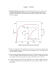### HS6X (HIGH-STRENGTH 6000 SERIES ALLOY)*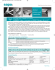### ATTENDEE INFORMATION REGISTRATION FEES (please circle selection) MPA/ASME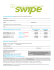### Environmental Policy Program Option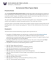### timetal 35a - PCC Energy Group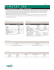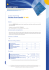### Size A3 Size A3 - Scana Booforge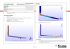### PH 840 ESTABLISHED RAIL INTERIOR PHENOLIC PREPREG ¬